Welcome to the Bartels Group of Companies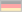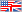Bartels :: Bartels AutoEngineer :: BAE Documentation :: User Language Programmer's Guide :: Conventions and Definitions :: Value Range Definitions
Bartels User Language - Programmer's Guide

# A.2 Value Range Definitions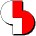User Language value ranges are defined for a series of index variable type elements and system function parameters. This section contains a complete survey over these definitions. In the description for the index variable types (see appendix B) and system functions (see appendix C) the herein defined value ranges are referenced by the corresponding designation of the value range.

## A.2.1 Standard Value Ranges (STD)

The following codes are valid for all caller types. I.e., they define valid value ranges for special elements of index variable types and/or system function parameters in the Schematic Editor, the Layout Editor, the Autorouter, the CAM Processor and the Chip Editor interpreter environments. The naming of the value range definitions emerges from the keyword `STD` and a continuous number.

#### STD1 - DDB Element Class:

```(-1) = Unknown/invalid DDB class
100 = Layout Plan
101 = Layout Part
102 = Layout Padstack
103 = Layout Pad
150 = Layout Paths
151 = Layout Editor Connectivity
160 = Layout DRC Parameters
200 = Physical Connection List
201 = Connection Assignments
202 = Backannotation Request
300 = Autorouter Data
301 = Autorouter Parameter
400 = BAE Character Font
401 = BAE Setup Data
402 = Parameter Setup Data
500 = Gerber Table
501 = Layout Color Table
502 = SCM Color Table
510 = IC Color Table
511 = GDS Structure
700 = BAE Messages
800 = SCM Sheet
801 = SCM Symbol
802 = SCM Marker
803 = SCM Label
850 = SCM Part List
900 = Logical Library Part
901 = Logical Connection List
902 = Physical Pin Info
903 = Packager Parameters
1000 = IC Layout
1001 = IC Cell
1002 = IC Pin
1050 = IC Paths
1051 = IC Connectivity
1052 = IC Autorouter Data
1200 = User Language Program
1201 = User Language Library
1400 = Design Rule
1401 = Design Rule List
1402 = Design Rule Source
4096 = SQL Structure Table Info
4097 = SQL Free Table Info
4352 = SQL user-defined Table
: = :
8191 = SQL user-defined Table
```

#### STD2 - Length Units:

`Length values are given in meters (unless otherwise mentioned).`

#### STD3 - Angle Units:

`Angle values are given in radians (unless otherwise mentioned).`

#### STD4 - Menu Item Numbers:

```[   0,  99] = Main menu items
[ 100, 199] = Menu 1 items
[ 200, 299] = Menu 2 items
[ 300, 399] = Menu 3 items
[ 400, 499] = Menu 4 items
[ 500, 599] = Menu 5 items
[ 600, 699] = Menu 6 items
[ 700, 799] = Menu 7 items
[ 800, 899] = Menu 8 items
[ 900, 999] = Menu 9 items
[1000,1099] = Menu 10 items
9003 = Menu Function Undo
9004 = Menu Function Redo
9005 = Close currently loaded element
9006 = Jump to Schematic Editor
9007 = Jump to Packager
9008 = Jump to Packager, run Packager and
jump to Schematic Editor if no Packager error occurred
9009 = Jump to Packager, run Packager and
jump to Layout Editor if no Packager error occurred
9010 = Jump to Layout Editor
9012 = Jump to Autorouter
9013 = Jump to CAM Processor
9014 = Jump to CAM View
9015 = BAE HighEnd/IC Design: Jump to IC Design Chip Editor
9016 = BAE HighEnd/IC Design: Jump to IC Design CIF View
9017 = BAE HighEnd/IC Design: Jump to IC Design GDS View
9018 = BAE HighEnd/IC Design: Jump to IC Design Cell Placer
9020 = Jump to BAE Setup
9021 = Jump to BAE Main Menu
9022 = BAE HighEnd: Start Schematic Editor instance
9023 = BAE HighEnd: Start Packager instance
9024 = BAE HighEnd: Start and run Packager instance
9025 = BAE HighEnd: Start Layout Editor instance
9027 = BAE HighEnd: Start Autorouter instance
9028 = BAE HighEnd: Start CAM Processor instance
9029 = BAE HighEnd: Start CAM View instance
9030 = BAE HighEnd: Start IC Design Chip Editor instance
9031 = BAE HighEnd: Start IC Design CIF View instance
9032 = BAE HighEnd: Start IC Design GDS View instance
9033 = BAE HighEnd: Start IC Design Cell Placer instance
9035 = BAE HighEnd: Start BAE Main Menu instance
9036 = Menu function Exit
9038 = Menu function Help
9039 = Menu function Help to
9041 = Jump to Packager, run Packager and
jump to Chip Editor if no Packager error occurred
9042 = Cut to Clipboard
9043 = Copy to Clipboard
9044 = Paste from Clipboard
9048 = Start program and wait for its termination
9049 = Start program and return
```

Windows/Motif dialogs:

```       5000 = Schematic Editor: Display parameters dialog
5001 = Schematic Editor: General Schematic Editor parameters dialog
5002 = Schematic Editor: SCM Plot parameters dialog
5005 = Layout Editor: Display parameters dialog
5006 = Layout Editor: General Layout Editor parameters
5008 = Layout Editor: Copper fill parameters dialog
5010 = Layout Editor: Autoplacement parameters dialog
5000 = Autorouter: Display parameters dialog
5001 = Autorouter: General Autorouter parameters dialog
5002 = Autorouter: Automatic placement parameters dialog
5003 = Autorouter: Autorouting options dialog
5004 = Autorouter: Autorouting control parameters dialog
5005 = Autorouter: Autorouting strategy parameters dialog
5006 = Autorouter: Autorouting batch setup dialog
5000 = CAM Processor: Display parameters dialog
5001 = CAM Processor: Control plot parameters dialog
5002 = CAM Processor: Gerber photoplot parameters dialog
5003 = CAM Processor: Drilling data output parameters dialog
5004 = CAM Processor: General CAM/Plot parameters dialog
5000 = CAM View: Display parameters dialog
5001 = CAM View: General CAM View parameters dialog
```

Standard menu item numbers are calculated using the

`100 × main menu number + submenu number`

formula with numbering starting at zero. The main menu number 0 is reserved for the menu, i.e., the Undo and Redo functions must be called through menu numbers 9003 and/or 9004, respectively.

#### STD5 - Dialog Element Parameter Type:

```                     Parameter values:
0 = 0x000000 = String value
1 = 0x000001 = Boolean value (check box)
2 = 0x000002 = Integer value
3 = 0x000003 = Double value
Display element types:
4 = 0x000004 = Label/title text/string
5 = 0x000005 = Horizontal seperator graphic
6 = 0x000006 = Vertical seperator graphic
Multiple choice dialog element types:
7 = 0x000007 = Radio box first option
8 = 0x000008 = Radio box next option
9 = 0x000009 = Selection box base entry
10 = 0x00000A = Selection box next entry
65536 = 0x010000 = List box base entry
65537 = 0x010001 = List box next entry
Dialog button types:
11 = 0x00000B = Action button
12 = 0x00000C = OK button
13 = 0x00000D = Abort button
Special parameter type codes:
14 = 0x00000E = Dummy dialog element
983055 = 0x0F000F = Parameter type mask (for parameter type queries)
Numerical value types:
16 = 0x000010 = Signed numerical value type
32 = 0x000020 = Distance/length numerical value type
64 = 0x000040 = Rotation angle numerical value type
Value range checking parameter types:
128 = 0x000080 = Lower boundary checking parameter type
256 = 0x000100 = Upper boundary checking parameter type
512 = 0x000200 = Immediate lower boundary checking parameter type
1024 = 0x000400 = Immediate upper boundary checking parameter type
Miscellaneous parameter types:
2048 = 0x000800 = Empty string display field parameter type
4096 = 0x001000 = Bold typeface font parameter type
8192 = 0x002000 = Fixed width font parameter type
16384 = 0x004000 = Double click confirmation parameter type
32768 = 0x008000 = Edit disabled parameter type
1048576 = 0x100000 = Single click confirmation parameter type
```

#### STD6 - Interaction Mode:

```0 = Input interactive
1 = Input automatic
```

#### STD7 - Coordinate Display Mode:

```0 = Display/input in mm units (micrometer units in IC Design)
1 = Display/input in Inch units (mm units in IC Design)
```

#### STD8 - Grid Lock Flag:

```0 = Grid unlocked
1 = Grid locked
```

#### STD9 - Angle Lock Flag:

```0 = Angle unlocked
1 = Angle locked
```

#### STD10 - Workspace Flag:

```0 = Element/object is out of workspace
1 = Element/object is in workspace
```

#### STD11 - Fixed Flag:

```0 = Element/object is unfixed
1 = Element/object is fixed
```

#### STD12 - Element Glued Mode:

```0 = Element/object is not glued
2 = Element/object is glued
```

#### STD13 - Group Flag:

```0 = Element/object is not selected to group
1 = Element/object is selected to group
2 = Toggle group selection status
```

#### STD14 - Mirror Mode:

```0 = Element/object is not mirrored
1 = Element/object is mirrored
```

#### STD15 - Polygon Point Type:

```0 = Normal point
1 = Left arc center point
2 = Right arc center point
```

#### STD16 - Macro Completion Status:

```xxxxxxx1 = Macro is completed (bit mask)
xxxxxx1x = Macro is missing (bit mask)
else = internal
```

#### STD17 - Mouse Button Key Codes:

```0 = Keyboard Input
1 = Left Mouse Button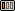2 = Middle Mouse Button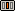3 = Right Mouse Button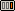```

#### STD18 - Color Codes:

```  0 = Black (no color)
1 = Blue
2 = Green
3 = Cyan
4 = Red
5 = Magenta
6 = Brown
7 = Light Gray
8 = Dark Gray
9 = Light Blue
10 = Light Green
11 = Light Cyan
12 = Light Red
13 = Light Magenta
14 = Yellow
15 = White
-1 = Black faded-out
-2 = Blue faded-out
-3 = Green faded-out
-4 = Cyan faded-out
-5 = Red faded-out
-6 = Magenta faded-out
-7 = Brown faded-out
-8 = Light Gray faded-out
-9 = Dark Gray faded-out
-10 = Light Blue faded-out
-11 = Light Green faded-out
-12 = Light Cyan faded-out
-13 = Light Red faded-out
-14 = Light Magenta faded-out
-15 = Yellow faded-out
-16 = White faded-out
```

#### STD19 - Drawing Mode:

```0 = Replace
1 = Clear
2 = Set
3 = Complement
```

#### STD20 - Polygon Fill/Drawing Mode:

``` 0 = Closed line polygon
1 = Closed fill polygon
2 = Open line polygon
3 = Closed pattern fill polygon
+4 = Dashed polygon outline
+8 = Dotted polygon outline
```

#### STD21 - Interaction Item Store Mode:

```0 = Append interaction placeholder to interaction queue end
1 = Append automatic interaction to interaction queue end
2 = Insert interaction placeholder at interaction queue start
3 = Insert automatic interaction at interaction queue start
```

## A.2.2 Schematic Capture Value Ranges (CAP)

The following codes are valid for caller types CAP and SCM. I.e., they define valid value ranges for special elements of index variable types and/or system function parameters in the Schematic Editor interpreter environment. The naming of the value range definitions emerges from the keyword `CAP` and a continuous number.

#### CAP1 - Schematic Capture Text Mode:

```0 = Standard Text (bit mask to be combined with CAP7)
1 = Comment Text (bit mask to be combined with CAP7)
```

#### CAP2 - Schematic Capture Polygon Type:

```0 = Line Cosmetic
1 = Filled Cosmetic
2 = internal
3 = internal
4 = Connection Point
5 = Dotted Line
```

#### CAP3 - Schematic Capture Figure Element Type:

```1 = Polygon
2 = Connection
3 = Named Reference
4 = internal
5 = Text
6 = Name pattern
7 = internal
8 = Polygon corner pick
9 = Named symbol reference pick
10 = Named label reference pick
11 = Named reference attribute pick
```

#### CAP4 - Schematic Capture Pool Element Type:

```  -1 = Unknown/undefined Element
1 = Element Type Macro (C_MACRO)
3 = Element Type Named Reference (C_NREF)
6 = Element Type Attribute Value (C_ATTRIBUTE.VALUE)
7 = Element Type Attribute Name (C_ATTRIBUTE.NAME)
16 = Element Type Polygon (C_POLY)
17 = Element Type Text (C_TEXT)
18 = Element Type Connection Segment List (C_CONBASE)
19 = Element Type Bus Tap (C_BUSTAP)
20 = Element Type Part Name Pattern (C_MACRO.PNAMEPAT)
32 = Element Type Font Name
else = internal
```

#### CAP5 - Schematic Tag Symbol/Label Mode:

```1 = Standard Symbol / Standard Label
2 = Virtual Tag Symbol
3 = Netlist Tag Symbol / Net Attribute Label
```

#### CAP6 - Schematic Capture Tag Pin Type:

```0 = Standard Pin or Label
1 = Symbol Destination Tag Pin
2 = Pin Destination Tag Pin
3 = Net Destination Tag Pin
4 = Net Pin Destination Tag Pin
3 = Net Area Destination Tag Pin
```

#### CAP7 - Schematic Capture Text Style Bit Mask:

```xxxx000x = Standard text style (no frame)
xxxxxx1x = Frame 1; surrounding box at 1/8 text height distance from text
xxxxx1xx = Frame 2; surrounding box at 1/4 text height distance from text
xxxx1xxx = Open frame(s); surrounding box(es) open at text origin side
xxx1xxxx = No text rotation
xx1xxxxx = Horizontally centered text flag
x1xxxxxx = Vertically centered text flag
1xxxxxxx = Right-aligned text flag
```

## A.2.3 Schematic Editor Ranges (SCM)

The following codes are valid for caller type SCM. I.e., they define valid value ranges for special system function parameters in the Schematic Editor interpreter environment. The naming of the value range definitions emerges from the keyword `SCM` and a continuous number.

#### SCM1 - SCM Display Item Types:

```(-16384) = -0x4000 = Invalid Display Item
0 = Documentation
1 = Connections
2 = Symbols
3 = Markers
4 = Symbol Borders
5 = internal
6 = internal
7 = Connect Area
8 = Work Area
9 = Origin
10 = Highlight
11 = Commentary Text
12 = Tag Symbol
13 = Tag Link
14 = Variant Attribute
15 = Plot disabled (elements which are excluded from plot outputs)
```

#### SCM2 - Schematic Display Item Class Level Bit Mask:

```xxxxxxxxxxx1 = Display item on plan level
xxxxxxxxxx1x = Display item on symbol level
xxxxxxxxx1xx = Display item on label level
xxxxxxxx1xxx = Display item on marker level
```

## A.2.4 Layout Value Ranges (LAY)

The following codes are valid for caller types LAY, GED, AR and CAM. I.e., they define valid value ranges for special elements of index variable types and/or system function parameters in the Layout Editor, the Autorouter and the CAM Processor interpreter environments. The naming of the value range definitions emerges from the keyword `LAY` and a continuous number.

#### LAY1 - Layout Layer Number:

```(-16384) = -0x4000 = Invalid Layer
(-6) = Inside Signal Layers
(-5) = Part Side Layer
(-4) = Unroutes Layer
(-3) = Part Plan Layer
(-2) = Board Outline Layer
(-1) = All Signal Layers
0 = Signal Layer 1
1 = Signal Layer 2
2 = Signal Layer 3
: = Signal Layer :
99 = Signal Layer 100
768 = 0x300 = Power Layer 1
769 = 0x301 = Power Layer 2
770 = 0x302 = Power Layer 3
: =     : = Power Layer :
777 = 0x309 = Power Layer 10
778 = 0x30A = Power Layer 11
779 = 0x30B = Power Layer 12
1024 = 0x400 = Documentary Layer 1 Side 1
1025 = 0x401 = Documentary Layer 1 Side 2
1026 = 0x402 = Documentary Layer 1 Both Sides
1040 = 0x410 = Documentary Layer 2 Side 1
1041 = 0x411 = Documentary Layer 2 Side 2
1042 = 0x412 = Documentary Layer 2 Both Sides
: =     : = Documentary Layer :
1168 = 0x490 = Documentary Layer 10 Side 1
1169 = 0x491 = Documentary Layer 10 Side 2
1170 = 0x492 = Documentary Layer 10 Both Sides
1184 = 0x4A0 = Documentary Layer 11 Side 1
1185 = 0x4A1 = Documentary Layer 11 Side 2
1186 = 0x4A2 = Documentary Layer 11 Both Sides
1200 = 0x4B0 = Documentary Layer 12 Side 1
1201 = 0x4B1 = Documentary Layer 12 Side 2
1202 = 0x4B2 = Documentary Layer 12 Both Sides
: =     : = Documentary Layer :
2592 = 0xA20 = Documentary Layer 99 Side 1
2593 = 0xA21 = Documentary Layer 99 Side 2
2594 = 0xA22 = Documentary Layer 99 Both Sides
2608 = 0xA30 = Documentary Layer 100 Side 1
2609 = 0xA31 = Documentary Layer 100 Side 2
2610 = 0xA32 = Documentary Layer 100 Both Sides
```

#### LAY2 - Layout Text Mode:

```0 = Physical
1 = Logical
2 = Norotate
```

#### LAY3 - Layout Polygon Mirror Visibility:

```0 = Visible always
1 = Visible if not mirrored
2 = Visible if mirrored
17 = Fixed visible if not mirrored
18 = Fixed visible if mirrored
```

#### LAY4 - Layout Polygon Type:

```1 = Copper
2 = Forbidden Area
3 = Border
4 = Connected Copper
5 = Line Cosmetic
6 = Filled Cosmetic
7 = Copperfill Workarea
8 = Hatched Copper
9 = Split Power Plane Area
```

#### LAY5 - Layout Drilling Class:

``` 0 = `-` (standard default)
1 = `A` (standard/unmirrored)
2 = `B` (standard/unmirrored)
3 = `C` (standard/unmirrored)
4 = `D` (standard/unmirrored)
5 = `E` (standard/unmirrored)
6 = `F` (standard/unmirrored)
7 = `G` (standard/unmirrored)
8 = `H` (standard/unmirrored)
9 = `I` (standard/unmirrored)
10 = `J` (standard/unmirrored)
11 = `K` (standard/unmirrored)
12 = `L` (standard/unmirrored)
13 = `M` (standard/unmirrored)
14 = `N` (standard/unmirrored)
15 = `O` (standard/unmirrored)
16 = `P` (standard/unmirrored)
17 = `Q` (standard/unmirrored)
18 = `R` (standard/unmirrored)
19 = `S` (standard/unmirrored)
20 = `T` (standard/unmirrored)
21 = `U` (standard/unmirrored)
22 = `V` (standard/unmirrored)
23 = `W` (standard/unmirrored)
24 = `X` (standard/unmirrored)
25 = `Y` (standard/unmirrored)
26 = `Z` (standard/unmirrored)
0x0080 =  0 × 256 + 128 = `-` (mirrored default)
0x0180 =  1 × 256 + 128 = `A` (mirrored)
0x0280 =  2 × 256 + 128 = `B` (mirrored)
0x0380 =  3 × 256 + 128 = `C` (mirrored)
0x0480 =  4 × 256 + 128 = `D` (mirrored)
0x0580 =  5 × 256 + 128 = `E` (mirrored)
0x0680 =  6 × 256 + 128 = `F` (mirrored)
0x0780 =  7 × 256 + 128 = `G` (mirrored)
0x0880 =  8 × 256 + 128 = `H` (mirrored)
0x0980 =  9 × 256 + 128 = `I` (mirrored)
0x0A80 = 10 × 256 + 128 = `J` (mirrored)
0x0B80 = 11 × 256 + 128 = `K` (mirrored)
0x0C80 = 12 × 256 + 128 = `L` (mirrored)
0x0D80 = 13 × 256 + 128 = `M` (mirrored)
0x0E80 = 14 × 256 + 128 = `N` (mirrored)
0x0F80 = 15 × 256 + 128 = `O` (mirrored)
0x1080 = 16 × 256 + 128 = `P` (mirrored)
0x1180 = 17 × 256 + 128 = `Q` (mirrored)
0x1280 = 18 × 256 + 128 = `R` (mirrored)
0x1380 = 19 × 256 + 128 = `S` (mirrored)
0x1480 = 20 × 256 + 128 = `T` (mirrored)
0x1580 = 21 × 256 + 128 = `U` (mirrored)
0x1680 = 22 × 256 + 128 = `V` (mirrored)
0x1780 = 23 × 256 + 128 = `W` (mirrored)
0x1880 = 24 × 256 + 128 = `X` (mirrored)
0x1980 = 25 × 256 + 128 = `Y` (mirrored)
0x1A80 = 26 × 256 + 128 = `Z` (mirrored)
```

Mirrored drill classes are intended for mirrored blind and buried via padstack definitions. Standard and mirrored drill class codes can be combined into a single drill class specification by adding or bit-or-ing the desired drill classes as in

```6 + 0x0880
```

for standard drill class `F` (6) and mirrored drill class `H` (0x0880).

#### LAY6 - Layout Figure Element Type:

```1 = Polygon
2 = Path
3 = Named Reference
4 = Unnamed Reference
5 = Text
6 = Drill
7 = internal
8 = Polygon corner pick
9 = Trace corner/pick
10 = Fill area polygon pick
```

#### LAY7 - Layout Level Type:

```>= 0 = Single Tree Level
(-1) = Multiple Trees Level (Short Circuit)
(-2) = Changed Level, no Tree
(-3) = Assigned Level (internal)
```

#### LAY8 - Layout Pool Element Type:

```  -1 = Unknown/undefined Element
1 = Element Type Macro (L_MACRO)
5 = Element Type Unnamed Reference (L_UREF)
6 = Element Type Named Reference (L_NREF)
8 = Element Type Attribute Value (L_ATTRIBUTE.VALUE)
9 = Element Type Attribute Name (L_ATTRIBUTE.NAME)
16 = Element Type Polygon (L_POLY)
17 = Element Type Path (L_LINE)
18 = Element Type Text (L_TEXT)
19 = Element Type Drill (L_DRILL)
21 = Element Type Hatched Polygon Path
32 = Element Type Part Side Layer
33 = Element Type Power Layer Net
34 = Element Type DRC Parameter
35 = Element Type Font Name
48 = Element Type DRC Error Marker (L_DRCERROR)
else = internal
```

#### LAY9 - Layout Display Item Types (additional to LAY1):

``` (-7) = Drill Holes (transfered to classes '-', 'A'-'Z')
(-8) = Work Area
(-9) = Origin
(-10) = Error
(-11) = Highlight
(-12) = Drill Holes Class '-'
(-13) = Drill Holes Class 'A'
: = :
(-38) = Drill Holes Class 'Z'
(-39) = Fixed
(-40) = Glued
```

#### LAY10 - Layout Mincon Function Type:

```0 = Mincon Off
1 = Pins Horizontal
2 = Pins Vertical
3 = Pins Horizontal+Vertical Sum
4 = Pins Airline
5 = Corners Horizontal
6 = Corners Vertical
7 = Corners Horizontal+Vertical Sum
8 = Corners Airline
```

#### LAY11 - Layout Input Item Type:

```>= 0 = Pool element input
(-1) = Rubberband input
(-2) = Window input
(-3) = Circle center input
(-4) = Anti clockwise arc input
(-5) = Clockwise arc input
(-6) = Segment move input
(-7) = Segment cut input
(-8) = Segment marker input
(-9) = Rubberband input type 2
```

#### LAY12 - Layout Variant Visibility:

```0-99 = Visible for given variant number
100  = Visible for all variants
101  = Visible for unplaced variant
```

#### LAY13 - Layout DRC Error Display Mode:

```1 = DRC copper distance violation
2 = DRC documentary layer forbidden area violation
3 = DRC documentary layer forbidden area height violation
4 = DRC HF design rule violation
5 = DRC invalid dropped polygon range
7 = DRC part side violation
8 = Fill polygon error hint
|65536 = DRC error marked as hidden
```

#### LAY14 - Layout Text Style Bit Mask:

```xxx000xxxxxx = Standard text style (no frame)
xxxxx1xxxxxx = Frame 1; surrounding box at 1/8 text height distance from text
xxxx1xxxxxxx = Frame 2; surrounding box at 1/4 text height distance from text
xxx1xxxxxxxx = Open frame(s); surrounding box(es) open at text origin side
xx1xxxxxxxxx = Horizontally centered text flag
x1xxxxxxxxxx = Vertically centered text flag
1xxxxxxxxxxx = Right-aligned text flag
```

#### LAY15 - Layout Display Item Class Level Bit Mask:

```xxxxxxxxxxx1 = Display item on layout level
xxxxxxxxxx1x = Display item on part level
xxxxxxxxx1xx = Display item on padstack level
xxxxxxxx1xxx = Display item on pad level
```

## A.2.5 CAM Processor Value Ranges (CAM)

The following codes are valid for caller type CAM. I.e., they define valid value ranges for special system function parameters in the CAM Processor interpreter environment. The naming of the value range definitions emerges from the keyword `CAM` and a continuous number.

#### CAM1 - CAM Processor Mirror Mode:

```0 = Mirroring off
1 = Mirroring on
2 = X-Backside (Mirroring off)
3 = X-Backside (Mirroring on)
4 = Y-Backside (Mirroring off)
5 = Y-Backside (Mirroring on)
```

#### CAM2 - CAM Processor Gerber Output Plotter Unit Length (STD2):

```0.0000254     = 2.3 Inch Format
0.00000254    = 2.4 Inch Format
0.000000254   = 2.5 Inch Format
0.0000000254  = 2.6 Inch Format
0.00000000254 = 2.7 Inch Format
or any other value greater than 0.00000000053
```

#### CAM3 - CAM Processor Gerber Output Format:

```     0 = 2.3 Inch Format
1 = 2.4 Inch Format
2 = 2.5 Inch Format
3 = 2.6 Inch Format
4 = 3.3 Metric Format
5 = 3.4 Metric Format
6 = 3.5 Metric Format
7 = 3.6 Metric Format
```

#### CAM4 - CAM Processor HP-GL Plot Pen Number:

```     1 = Enabled Pen 1
2 = Enabled Pen 2
3 = Enabled Pen 3
4 = Enabled Pen 4
5 = Enabled Pen 5
6 = Enabled Pen 6
7 = Enabled Pen 7
8 = Enabled Pen 8
9 = Enabled Pen 9
10 = Enabled Pen 10
: = Enabled Pen :
100 = Enabled Pen 100
(-1) = Disabled/Invalid Pen Number
(-2) = Disabled Pen 1
(-3) = Disabled Pen 2
(-4) = Disabled Pen 3
(-5) = Disabled Pen 4
(-6) = Disabled Pen 5
(-7) = Disabled Pen 6
(-8) = Disabled Pen 7
(-9) = Disabled Pen 8
(-10) = Disabled Pen 9
(-11) = Disabled Pen 10
:  = Disabled Pen :
(-101) = Disabled Pen 100
```

## A.2.6 IC Design Value Ranges (ICD)

The following codes are valid for caller types ICD and CED. I.e., they define valid value ranges for special elements of index variable types and/or system function parameters in the Chip Editor interpreter environment. The naming of the value range definitions emerges from the keyword `ICD` and a continuous number.

#### ICD1 - IC Design Layer Number:

```(-16384) = -0x4000 = Invalid Layer
(-3) = Unroutes Layer
(-2) = Border Layer
(-1) = All Layers
0 = IC Layer 1
1 = IC Layer 2
2 = IC Layer 3
: = IC Layer :
99 = IC Layer 100
```

#### ICD2 - IC Design Text Mode:

```0 = Physical
1 = Logical
2 = Norotate
```

#### ICD3 - IC Design Polygon Mirror Visibility:

```0 = Visible always
1 = Visible if not mirrored
2 = Visible if mirrored
```

#### ICD4 - IC Design Polygon Type:

```1 = Active Area
2 = Forbidden Area
3 = Line Cosmetic
4 = Border
```

#### ICD5 - IC Design Figure List Element Type:

```1 = Polygon
2 = Path
3 = Named Reference
4 = Unnamed Reference
5 = Text
6 = internal
7 = Polygon corner pick
8 = Trace corner/pick
```

#### ICD6 - IC Design Level Type:

```>= 0 = Single Tree Level
(-1) = Multiple Trees Level (Short Circuit)
(-2) = Changed Level, no Tree
(-3) = Assigned Level (internal)
```

#### ICD7 - IC Design Pool Element Type:

```  -1 = Unknown/undefined Element
1 = Element Type Macro (I_MACRO)
2 = Element Type Unnamed Reference (I_UREF)
3 = Element Type Named Reference (I_NREF)
6 = Element Type Attribute Value (I_ATTRIBUTE.VALUE)
7 = Element Type Attribute Name (I_ATTRIBUTE.NAME)
16 = Element Type Polygon (I_POLY)
17 = Element Type Path (I_LINE)
18 = Element Type Text (I_TEXT)
else = internal
```

#### ICD8 - IC Design Display Item Types (additional to ICD1):

```(-6) = Work Area
(-7) = Origin
(-8) = Error
(-9) = Highlight
```

#### ICD9 - IC Design Layer Display Mode Bit Mask:

```xxxxx00 = Display layer items with outline
xxxxx01 = Display layer items with filled area
xxxxx10 = Display layer items with dash outline
xxxxx11 = Display layer items with pattern filled area
00000xx = Display layer items with pattern 0 filled area
.....xx = Display layer items with pattern : filled area
11111xx = Display layer items with pattern 31 filled area
```

#### ICD10 - IC Design Mincon Function Type:

```0 = Mincon Off
1 = Pins Horizontal
2 = Pins Vertical
3 = Pins Horizontal+Vertical Sum
4 = Pins Airline
5 = Corners Horizontal
6 = Corners Vertical
7 = Corners Horizontal+Vertical Sum
8 = Corners Airline
```
 Bartels :: Bartels AutoEngineer :: BAE Documentation :: User Language Programmer's Guide :: Conventions and Definitions :: Value Range Definitions

Value Range Definitions
© 1985-2019 Oliver Bartels F+E • Updated: 25 May 2013, 15:34 [UTC]© 1985-2019 Oliver Bartels F+E Bartels Homepage Contact and Corporate InfoWeb Development by Baumeister Mediasoft Engineering